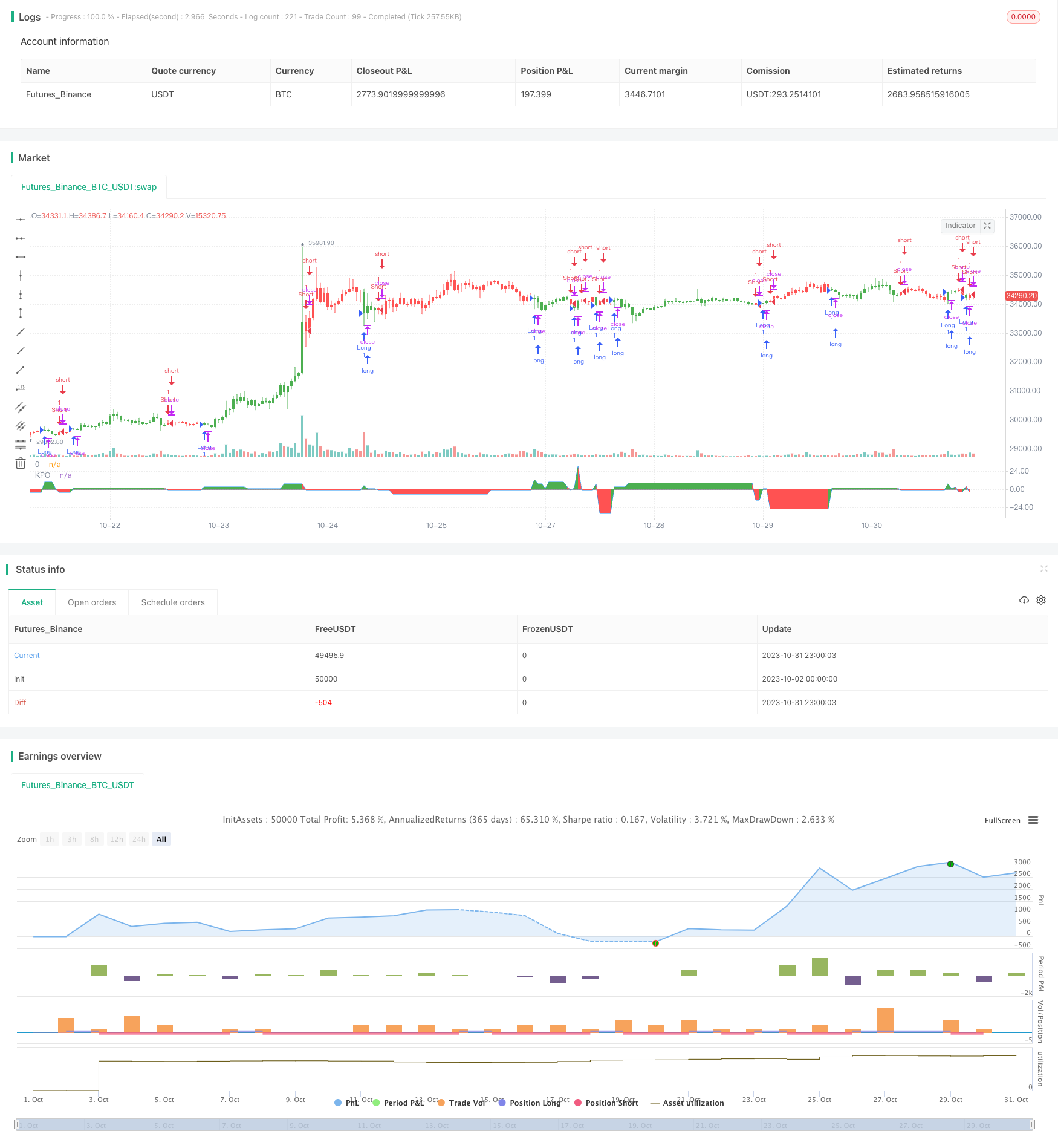# Prime Number Oscillator Trading Strategy

Author: ChaoZhang, Date: 2023-11-02 14:42:22
Tags:## Overview

This strategy uses the Prime Number Oscillator indicator to determine market trends and construct long/short positions accordingly. The PNO calculates the difference between the nearest prime number to price and the price itself, with positive values indicating bullish trends and negative values indicating bearish trends. The strategy can capture hidden trend information during price oscillations and provide guidance for breakout trading.

## Strategy Logic

The strategy first defines a PrimeNumberOscillator function that takes price and allowedPercent as parameters. The function searches for the nearest prime number to price within the allowedPercent range and returns their difference. A positive difference indicates bullish and a negative difference indicates bearish.

In the strategy, the PrimeNumberOscillator function is called to compute the xPNO value. The position direction is determined by the sign of xPNO and multiplied by the reverseFactor to get the final trade direction. Long/short positions are opened based on the direction.

The strategy mainly relies on the PNO indicator for trend direction. The indicator itself is quite crude and needs to be combined with other factors for signal verification. But it is based on mathematical principles and can provide some objective guidance.

• Based on mathematical principles, relatively objective
• Can identify trends hidden in oscillations
• Flexible parameter tuning for sensitivity adjustment
• Simple to implement, easy to understand and optimize

## Risk Analysis

• PNO itself is crude, prone to multiple false signals
• Needs verification from other technical indicators, cannot be used alone
• Careful parameter selection needed, too large or small will fail
• High trading frequency, position sizing needs control

## Optimization Directions

• Add filters like moving average, RSI for signal verification
• Implement stop loss to limit downside risk
• Dynamically adjust allowedPercent based on market conditions
• Optimize position sizing through volatility and other metrics

## Conclusion

The strategy determines trend direction based on prime number oscillation principles, with simple logic and implementation. But PNO has limitations that require cautious use. Combining other technical indicators to verify signals and control risk is needed. As a typical representative of mathematical trading strategies, it has reference value for study and research.

```/*backtest
start: 2023-10-02 00:00:00
end: 2023-11-01 00:00:00
period: 1h
basePeriod: 15m
exchanges: [{"eid":"Futures_Binance","currency":"BTC_USDT"}]
*/

//@version=2
////////////////////////////////////////////////////////////
//  Copyright by HPotter v1.0 29/03/2018
// Determining market trends has become a science even though a high number or people
// still believe it’s a gambling game. Mathematicians, technicians, brokers and investors
// have worked together in developing quite several indicators to help them better understand
// and forecast market movements.
//
// Developed by Modulus Financial Engineering Inc., the prime number oscillator indicates the
// nearest prime number, be it at the top or the bottom of the series, and outlines the
// difference between that prime number and the respective series.
//
// You can change long to short in the Input Settings
// WARNING:
//  - For purpose educate only
//  - This script to change bars colors.
////////////////////////////////////////////////////////////
res = 0
res1 = 0
res2 = 0
for j = price to price + (price * percent / 100)
res1 := j
for i = 2 to sqrt(price)
res1 := iff(j % i == 0 , 0, j)
if res1 == 0
break
if res1 > 0
break
for j = price to price - (price * percent / 100)
res2 := j
for i = 2 to sqrt(price)
res2 := iff(j % i == 0 , 0, j)
if res2 == 0
break
if res2 > 0
break
res := iff(res1 - price < price - res2,  res1 - price, res2 - price)
res := iff(res == 0, res, res)
res

strategy(title="Prime Number Oscillator Backtest")
percent = input(5, minval=0.01, step = 0.01, title="Tolerance Percentage")
pos = iff(xPNO > 0, 1,
iff(xPNO < 0, -1, nz(pos, 0)))
possig = iff(reverse and pos == 1, -1,
iff(reverse and pos == -1, 1, pos))
if (possig == 1)
strategy.entry("Long", strategy.long)
if (possig == -1)
strategy.entry("Short", strategy.short)
barcolor(possig == -1 ? red: possig == 1 ? green : blue )
clr = iff(xPNO > 0, green, red)
p1 = plot(xPNO, color=blue, title="KPO")
p2 = plot(0, color=black, title="0")
fill(p1,p2,color=clr)
```

More